Courses

# Mole Concept JEE Notes | EduRev

## Class 11: Mole Concept JEE Notes | EduRev

The document Mole Concept JEE Notes | EduRev is a part of Class 11 category.
All you need of Class 11 at this link: Class 11

Mole

A mole is an amount unit similar to familiar units like pair, dozen, gross, etc.
It provides a specific measure of the number of atoms or molecules in a bulk sample of matter.

One mole is defined as the amount of substance containing the same number of discrete entities (atoms, molecules, ions, etc.) as the number of atoms in a sample of pure 12C weighing exactly 12 g.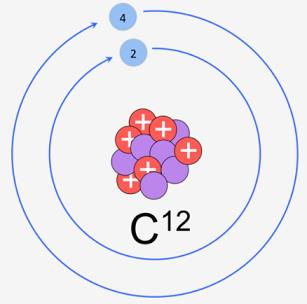C-12 isotope

One Latin connotation for the word “mole” is “large mass” or “bulk,” which is consistent with its use as the name for this unit.
The mole provides a link between an easily measured macroscopic property, bulk mass, and an extremely important fundamental property, the number of atoms, molecules, and so forth.

Q. In order to determine this number precisely, the mass of a carbon–12 atom was determined by a mass spectrometer and found to be equal to 1.992648 × 10–23 g. Knowing that one mole of carbon weighs 12 g, the number of atoms in it
Ans.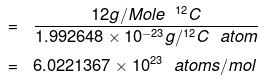The number of entities composing a mole has been experimentally determined to be 6.0221367 × 1023, a fundamental constant named Avogadro’s number (NA) or the Avogadro constant in honor of Italian scientist Amedeo Avogadro.
This constant is properly reported with an explicit unit of “per mole,” a conveniently rounded version being 6.022×1023/mol.
It may be emphasized that the mole of a substance always contains the same number of entities, no matter what the substance may be.

1 mole = collection of 6.02 × 1023 species
6.02 × 1023 = NA = Avogadro's No.

1 mole of atoms is also termed as 1 gm-atom, 1 mole of ions is termed as
1 gm-ion and 1 mole of a molecule termed as 1 gm-molecule.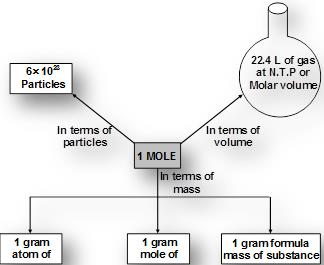Mole in different units

Methods of Calculations of Mole

(a) If no. of some species is given, then no. of moles = Given no./NA
(b) If weight of a given species is given, then no of moles = Given wt/Atomic wt. (for atoms)
or = Given wt./Molecular wt. (for molecules)
(c) If volume of a gas is given along with its temperature (T) and pressure (P)
use n = PV/RT
where R = 0.0821 lit-atm/mol-K (when P is in atmosphere and V is in litre.)

1 mole of any gas at STP (0°C & 1 bar) occupies 22.7 litre.
1 mole of any gas at STP (0°C & 1 atm) occupies 22.4 litre.

A mole of any substance is related to:
(a) Number of particles
(b) Mass of a substance
(c) Volume of the gaseous substance

Mole-Particle Relationship

• A mole is a collection of 6.023 × 1023 particles, ions, atoms etc.
• Avogadro Number (NA): The number of carbon atoms present in one gram-atom (1-mole atom) of C–12 isotope is called Avogadro’s number.
• One gram-atom (12 grams) of C–12 contains 6.02 × 1023 atoms. Thus the numerical value of Avogadro’s number (NA) is 6.02 × 1023 per mol.
• It should be noted that 1 a.m.u.= 1/12 th of mass of a Carbon-12 atom.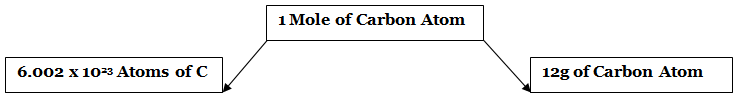(i) 6.023 × 1023 atoms of Na constitute one-mole atom of Na.
(ii) 6.023 × 1023 molecules of oxygen constitutes 1 mole of oxygen molecules.
(iii) 6.023 × 1023 electrons constitute one mole of electrons.
(iv) No. of moles = Number of particles/6.023 × 1023

Mole-Weight Relationship

• One mole of every substance weighs equal to the gram atomic weight of the substance or to the gram molecular weight of the substance.
Example:
(i) 1 mole of sodium weighs 23 g of Na.
(ii) 1 mole of CaCO3 weighs 100 g.
Number of moles = Mass of substance in grams/Mass of weight in grams

Mole-Volume Relationship

• One mole of every gas occupies 22.4 lit. of volume at STP i.e. 1 mole of O2 occupies 22400 ml of volume at STP.
• 1 mole of He occupies 22400 ml of volume at STP.
• Number of moles = 'V' of gas in litres at STP/22.4

Important Relations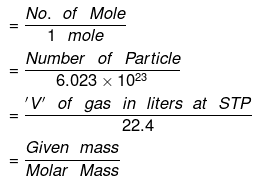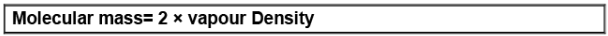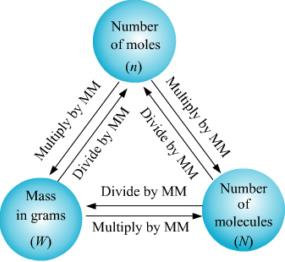MM: Molecular Mass

Elemental Analysis

• For 'n' mole of a compound (C3H7O2)
• Moles of C = 3n
• Moles of H = 7n
• Moles of O = 2n

Example 1. Find the weight of water present in 1.61 g of Na2SO410H2O
Solution. Moles of Na2SO4.10H2O =  0.005
Moles of water = 10 × moles of Na2SO4. 10H2O = 10 × 0.05 = 0.05
wt. of water = 0.5 × 18 = 0.9 gm

Example 2. A molecule of a compound has 13 carbon atoms, 12 hydrogen atom, 3 oxygen atoms and 3.02 × 10-23 gm of other element. Find the molecular wt. of compound.
Solution. Wt. of the 1 molecule of a compound = 13 × 12 × 3 × 3.02 × 10-23
= 234.18 / NA = 234 amu.

Example 3. Calculate the number of moles of electron present in 1 kg of it. (me = 9.1 × 10–31 kg)
Solution. Number of electrons in 1 kg = 1 kg/9.1 × 10-31 kg
Number of moles = Number of particles/6.023 × 1023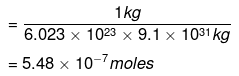Example 4. What will be the number of moles in 13.5 g of SO2Cl2?
Solution. Molecular mass of SO2Cl2 = 32 + 32 + 71 = 135 g mol–1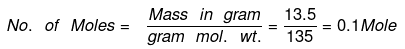Example 5. What will be the number of moles of oxygen in one litre of air containing 21% oxygen by volume under STP conditions?
Solution. 100 ml of air at STP contains 21 ml of O2
1000 ml of air at STP contains = 21/100 × 1000 = 210 ml O2
∴ No. of moles = Volume in ml under STP conditions/22400 litre
= 210/22400 = 9.38 × 10-3 mole.

Example 6. Calculate the mass of (i) an atom of silver (ii) a molecule of carbon dioxide.
Solution.
(i) 1 mole of Ag atoms = 108 g
(∴ Atomic mass of silver = 108u)
= 6.022 × 1023 atoms
6.022 × 1023 atoms of silver have mass = 108g
∴ Mass of one atom of silver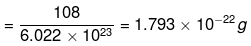(ii) 1 mole of CO2 = 44 g

(Molecular mass of CO2 = 1 x 12 + 2 x 16 = 44u)
= 6.022 × 1023 molecules
Thus, 6.022 × 1023 molecules of CO2 has mass = 44 g
∴ 1 molecule of CO2 has mass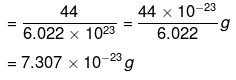Example 7. Calculate the number of molecules present
(i) In 34.20 grams of cane sugar (C12H22O11).
(ii) In one litre of water assuming that the density of water is 1 g/cm3.
(iii) In one drop of water having mass 0.05 g.
Solution.
(i) 1 mole of C12H22O11 = 342 g
[Molecular mass of cane sugar C12H22O11]
= 12 x 12 + 22 × 1 + 11 × 16 = 342 amu
= 6.022 × 1023 molecules
Now 342 g of cane sugar contain 6.022 × 1023 molecules.
∴ 34.2 g of cane sugar will contain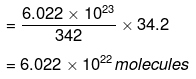(ii) 1 mole of water = 18 g = 6.022 × 1023 molecules.
Mass of 1 litre of water = Volume × density = 1000 × 1 = 1000 g
Now 18 g of water contains = 6.022 x 1023 molecules.
∴ 1000 g of water will contain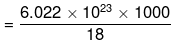= 3.346 x 1025 molecules
(iii) 1 mole of H2O = 18 g = 6.022 × 1023 molecules.
Mass of 1 drop of water = 0.05 g
Now 18 g of H2O contain = 6.022 × 1023 molecules.
∴ 0.05 g of H2O will contain =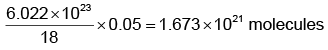Example 8. Calculate the number of moles in each of the following:
(i) 392 grams of sulphuric acid
(ii) 44.8 litres of carbon dioxide at STP
(iii) 6.022 × 1023 molecules of oxygen
(iv) 9.0 grams of aluminium
(v) 1 metric ton of iron (1 metric ton = 103 kg )
(vi) 7.9 mg of Ca
(vii) 65.5 mg of carbon

Solution.
(i) 1 mole of H2SO4 = 98 g

∵ Molecular mass of H2SO4 = 2 × 1 + 32 + 4 × 16 = 98u)
Thus 98 g of H2SO4 = 1 mole of H2SO4
∴ 392 g of H2SO4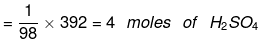(ii) 1 mole of CO2 = 22.4 litres at STP

i.e. 22.4 litres of CO2 at STP = 1 mole
∴ 44.8 litres of CO2 at STP
= 1/22.4 × 44.8
= 2 moles CO2

(iii) 1 mole of O2 molecules = 6.022 × 1023 molecules.

6.022 × 1023 molecules = 1 mole of oxygen molecules.

(iv) 1 mole of Al = 27 g of Al

(∴ Atomic mass of aluminium = 27 g)
i.e. 27 g of aluminium = 1 mole of Al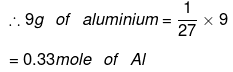(v) 1 metric ton of Fe = 103 kg = 106 g

1 mole of Fe = 56 g of Fe
∴ 106 g of Fe = 106/56 molecules
= 1.786 × 104 moles

(vi) 7.9 mg of Ca = 7.9 × 10−3 g of Ca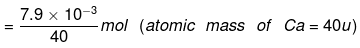(vii) 65.5 µg of C = 65.5 10-6 g of C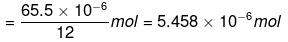Try Yourself!

Q.1. Find the weight of 6023 molecules of CO2 in grams.
Ans. 4.4 × 10–19 gm

Q.2. Calculate the total number of electrons present in 1.6 g of methane. (Molecular wt. of methane = 16 a.m.u.)
Ans. 6.023 × 1023 electrons

Q.3. 0.24 gm of a volatile substance upon vaporization gives 45 ml vapours at STP. What will be the vapour density of the substance?
Ans. 59.73

Q.4. What is the mass of carbon present in 0.5 mole of K4[Fe(CN)6]?
Ans. 36 gm

Q.5. Calculate how many methane molecules and how many hydrogen and carbon atoms are there in 25.0 g of methane?
Ans. 9.41 × 1023 CH4 molecules, 9.41 × 1023 carbon atoms and 37.64 × 1023 hydrogen atoms.

Q.6. One litre of gas at NTP weighs 1.97g. Find the molecular mass of gas.
Ans. 44.128 g

The document Mole Concept JEE Notes | EduRev is a part of Class 11 category.
All you need of Class 11 at this link: Class 11Use Code STAYHOME200 and get INR 200 additional OFF Use Coupon Code
All Tests, Videos & Notes of Class 11: Class 11

Track your progress, build streaks, highlight & save important lessons and more!

,

,

,

,

,

,

,

,

,

,

,

,

,

,

,

,

,

,

,

,

,

;